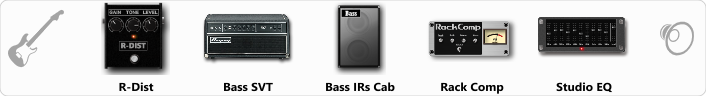# Bass #2

Discussion in 'ToneLib-GFX presets' started by Omega Station, Aug 11, 2019.

1. Bass #2

Preset name: OmSt Bass 2

You can find all the bass presets I made here: https://honestampsimreviews.com/preset-vault/ (under bass amp sims - Tonelib GFX)

Effects chain:Effect: "R-Dist" (Overdrive / Distortion), active - "yes"
"Drive" = 22
"Tone" = 41
"Level" = 42

Effect: "Bass SVT" (Amp simulators), active - "yes"
"Bass Ctrl" = Off
"Midrange" = 800 Hz
"Ultra Hi" = Off
"Bass" = 0
"Middle" = 2
"Treble" = 1
"Volume" = 70
"Level (dB)" = 0

Effect: "Bass IRs Cab" (Cabinets), active - "yes"
"Model" = Ampeg SVT 810e (8x10")
"Mic Position" = Middle
"Mic Distance" = Far
"Low Cut (Hz)" = 30
"Hi Cut (kHz)" = 7.0
"Mix" = 100
"Level (dB)" = 0

Effect: "Rack Comp" (Dynamics / Filter), active - "yes"
"Threshold (dB)" = -24
"Ratio" = 4
"Attack" = Fast
"Release (ms)" = 250
"Knee" = 0
"Level (dB)" = 2

Effect: "Studio EQ" (Dynamics / Filter), active - "yes"
"31 Hz" = 0
"62 Hz" = -2
"125 Hz" = -2
"250 Hz" = -3
"500 Hz" = -1
"1 kHz" = 1
"2 kHz" = 2
"4 kHz" = 1
"8 kHz" = -2
"16 kHz" = -4
"above 16 kHz" = 0
"Level (dB)" = 0

Note: You will need to download and install the ToneLib-GFX software to use the preset.

File size:
551 bytes
Views:
5,257